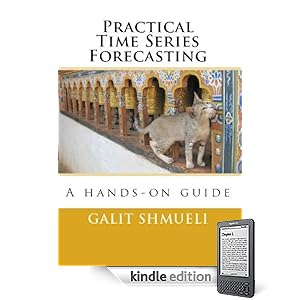## Monday, April 25, 2011

In business schools it is common to teach statistics courses using Microsoft Excel, due to its wide accessibility and the familiarity of business students with the software. There is a large debate regarding this practice, but at this point the reality is clear: the figure that I am familiar with is about 50% of basic stat courses in b-schools use Excel and 50% use statistical software such as Minitab or JMP.

Another trend is moving from offline software to "cloud computing" -- Software such as www.statcrunch.com offer basic stat functions in an online, collaborative, social-networky style.

Following the popularity of spreadsheet software and the cloud trend, I asked myself whether the free Google Spreadsheets can actually do the job. This is part of my endeavors to find free (or at least widely accessible) software for teaching basic concepts. While Google Spreadsheets does have quite an extensive function list, I discovered that its current computing is very limited. For example, computing binomial probabilities using the function BINOMDIST is limited to a sample size of about 130 (I did report this problem). Similarly, HYPGEOMDIST results in overflow errors for reasonably small sample and population sizes.

From the old days when we used to compute binomial probabilities manually, I am guessing that whoever programmed these functions forgot to use the tricks that avoid computing high factorials in n-choose-k type calculations...

## Saturday, April 16, 2011

### Moving Average chart in Excel: what is plotted?

In my recent book Practical Time Series Forecasting: A Practical Guide, I included an example of using Microsoft Excel's moving average plot to suppress monthly seasonality. This is done by creating a line plot of the series over time and then Add Trendline > Moving Average (see my post about suppressing seasonality). The purpose of adding the moving average trendline to a time plot is to better see a trend in the data, by suppressing seasonality.

A moving average with window width w means averaging across each set of w consecutive values. For visualizing a time series, we typically use a centered moving average with w = season.  In a centered moving average, the value of the moving average at time t (MAt) is computed by centering the window around time t and averaging across the w values within the window. For example, if we have daily data and we suspect a day-of-week effect, we can suppress it by a centered moving average with w=7, and then plotting the MA line.

An observant participant in my online course Forecasting discovered that Excel's moving average does not produce what we'd expect: Instead of averaging over a window that is centered around a time period of interest, it simply takes the average of the last w months (called a "trailing moving average"). While trailing moving averages are useful for forecasting, they are inferior for visualization, especially when the series has a trend. The reason is that the trailing moving average "lags behind". Look at the figure below, and you can see the difference between Excel's trailing moving average (black) and a centered moving average (red).

The fact that Excel produces a trailing moving average in the Trendline menu is quite disturbing and misleading. Even more disturbing is the documentation, which incorrectly describes the trailing MA that is produced:
"If Period is set to 2, for example, then the average of the first two data points is used as the first point in the moving average trendline. The average of the second and third data points is used as the second point in the trendline, and so on."
For more on moving averages, see here:

## Saturday, April 09, 2011

### Visualizing time series: suppressing one pattern to enhance another pattern

Visualizing a time series is an essential step in exploring its behavior. Statisticians think of a time series as a combination of four components: trend, seasonality, level and noise. All real-world series contain a level and noise, but not necessarily a trend and/or seasonality. It is important to determine whether trend and/or seasonality exist in a series in order to choose appropriate models and methods for descriptive or forecasting purposes. Hence, looking at a time plot,  typical questions include:
• is there a trend? if so, what type of function can approximate it? (linear, exponential, etc.) is the trend fixed throughout the period or does it change over time?
• is there seasonal behavior? if so, is seasonality additive or multiplicative? does seasonal behavior change over time?
Exploring such questions using time plots (line plots of the series over time) is enhanced by suppressing one type of pattern for better visualizing other patterns. For example, suppressing seasonality can make a trend more visible. Similarly, suppressing a trend can help see seasonal behavior. How do we suppress seasonality? Suppose that we have monthly data and there is apparent annual seasonality. To suppress seasonality (also called seasonal adjustment), we can1. Plot annual data (either annual averages or sums)
2. Plot a moving average (an average over a window of 12 months centered around each particular month)
3. Plot 12 separate series, one for each month (e.g., one series for January, another for February and so on)
4. Fit a model that captures monthly seasonality (e.g., a regression model with 11 monthly dummies) and look at the residual seriesAn example is shown in the Figure. The top left plot is the original series (showing monthly ridership on Amtrak trains). The bottom left panel shown a moving average line, suppressing seasonality and showing the trend. The top right panel shows a model that captures the seasonality. The lower left panel shows the residuals from the model, again enhancing the trend.

For further details and examples, see my recently published book Practical Time Series Forecasting: A Hands On Guide (available in soft-cover and as an eBook).